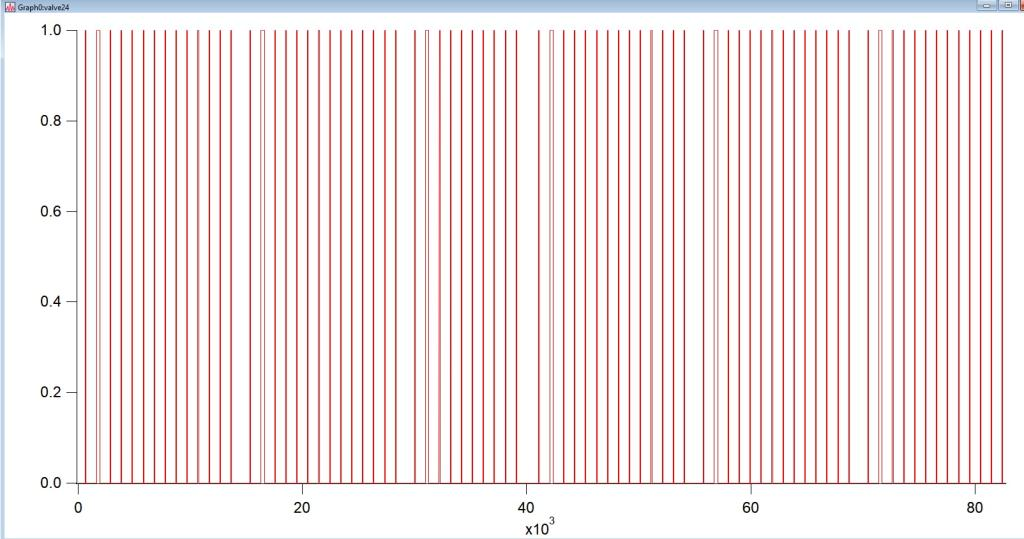# Averaging over select time windows across a timeseriesHello,

I have timeseries data that needs to be averaged over select time windows. The windows are defined by when the instrument valve was open (i.e. reading 1) . Kindly see the image below that shows the instances when the valve read 1. Ignore the x-axis (just index numbers). The valve only reads 1 and 0. Now let's say the data collected is wave1. What I want to output are average values from wave1 for every time window when the valve read 1, and all these values listed in a new 1D wave. So, if there are 80 instances of the valve opening to read 1, the new 1D wave will have 80 rows each row containing an average value for the particular window when the valve read 1.

Following this, I would like to introduce some lag in averaging, i.e. instead of averaging from right when the valve opens, I want to start averaging maybe 3 index numbers after, to avoid including any transition data points.

There may be an easy code for this but I'm kinda struggling.. would really appreciate some help!  Thanks a ton!

Sincerely,

PeeyushHi Andy,

I tried using extract but I'm running into the issue that it pulls out all values for which valve equals 1 across the timeseries. However, I need for it to pull out values for 1 valve opening window at a time. Then I can wavestats that to get average for the window. Is there a way to get around this?

Sincerely,

Peeyush

Here is an example:

make/o/n=1000 ddd=sin(x) > 0  // Make a square wave
Extract/O/INDX ddd, startIndexes, (ddd[p] == 1) && (p==0 || (ddd[p-1] == 0))

startIndexes now contains the point number of the first point of each interval during which ddd==1.

You can do something similar to get a wave containing the last point index in each interval:

Variable lastPointIndex = numpnts(ddd) - 1
Extract/O/INDX ddd, stopIndexes, (ddd[p] == 1) && (p!=lastPointIndex && (ddd[p+1] == 0))

Here is a quick and dirty function you could use to calculate the average over each interval:

Function avgWindow()
WAVE ddd, startIndexes, stopIndexes
Make/O/N=(numpnts(ddd)) timeSeriesData
MultiThread timeSeriesData = enoise(50) // Make some random data

Make/O/N=(numpnts(startIndexes)) avgWave
MultiThread avgWave = mean(timeSeriesData, x2pnt(ddd, startIndexes[p]), x2pnt(ddd, stopIndexes[p]))

End